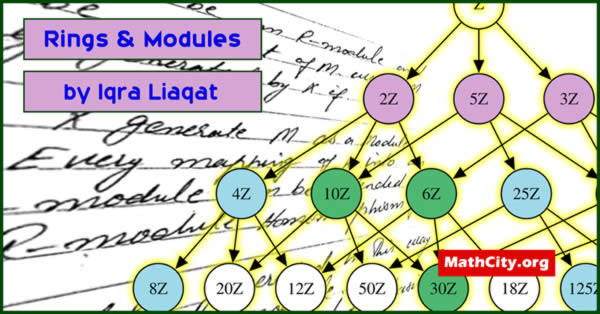# Rings & Modules by Ms. Iqra Liaqat

Ring is a mathematical structure with two operations. With one operation it is abelian group and with other operation it is semi-group with distributive law holds with first operation w.r.t the other operation. This concept is of pure in nature in mathematics. These advanced level notes are typically taken as an elective in a mathematics undergraduate degree.These notes are send by Ms. Iqra Liaqat. She generously provided these notes, and we appreciate the work she made to post them on MathCity.org.

 Name Rings & Modules Ms. Iqra Liaqat 140 pages PDF (see Software section for PDF Reader) 5.88 mB
• Groupoid
• Semi group
• Group
• Subgroup
• Ring
• Field
• Vector space
• Module
• Unital R module
• Module homomorphism
• Monomorphism
• Epimorphism
• Isomorphism
• Kernal of homomorphism
• Range of T
• Ring homomorphism
• Quotient Module
• First theorem of homomorphism
• Second isomorphism theorem
• Fundamental theorem of homomorphism
• Internal direct sum
• External direct sum
• Finitely generated module
• Cyclic module
• Torsion free module
• Torsion element
• Freely generated module
• Linearly independent & dependent set
• Polynomial ring
• Degree of polynomial
• Constant polynomial
• Remainder theorem
• Zeros of the polynomial
• Roots of the equation
• Factor theorem
• Existence theorem
• Gaussian integer and ring of Gaussian integer
• Prime
• Irreducible element
• Irreducible polynomial over field
• Monic polynomial
• Unique factorization domain (U.F.O)
• Principle ideal
• Principle ideal domain
• Euclidean domain
• Factorization domain

• notes/rings-and-modules-iqra-liaqat